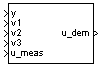# 3D Self-Conditioned [A(v),B(v),C(v),D(v)]

Implement gain-scheduled state-space controller in self-conditioned form depending on two scheduling parameters

•Libraries:
Aerospace Blockset / GNC / Control

## Description

The 3D Self-Conditioned [A(v),B(v),C(v),D(v)] block implements a gain-scheduled state-space controller as defined in Algorithms.

If the scheduling parameter inputs to the block go out of range, then they are clipped. The state-space matrices are not interpolated out of range.

The output from this block is the actuator demand, which you can input to an actuator block.

## Limitations

This block requires the Control System Toolbox™ license.

## Ports

### Input

expand all

Aircraft measurements, specified as a vector.

Data Types: `double`

First scheduling variable, specified as a vector, ordered according to the dimensions of the state-space matrices.

Data Types: `double`

Second scheduling variable, specified as a vector, ordered according to the dimensions of the state-space matrices.

Data Types: `double`

Third scheduling variable, specified as a vector, ordered according to the dimensions of the state-space matrices.

Data Types: `double`

Measured actuator position, specified as a vector.

Data Types: `double`

### Output

expand all

Actuator demands, specified as a vector.

Data Types: `double`

## Parameters

expand all

A-matrix of the state-space implementation. In the case of 3-D scheduling, the A-matrix should have five dimensions, the last three corresponding to scheduling variables v1, v2, and v3. For example, if the A-matrix corresponding to the first entry of v1, the first entry of v2, and the first entry of v3 is the identity matrix, then `A(:,:,1,1,1) = [1 0;0 1];`.

#### Programmatic Use

 Block Parameter: `A` Type: character vector Values: vector Default: `'A'`

B-matrix of the state-space implementation. In the case of 3-D scheduling, the B-matrix should have five dimensions, the last three corresponding to scheduling variables v1, v2, and v3. For example, if the B-matrix corresponding to the first entry of v1, the first entry of v2, and the first entry of v3 is the identity matrix, then `B(:,:,1,1,1) = [1 0;0 1];`.

#### Programmatic Use

 Block Parameter: `B` Type: character vector Values: vector Default: `'B'`

C-matrix of the state-space implementation. In the case of 3-D scheduling, the C-matrix should have five dimensions, the last three corresponding to scheduling variables v1, v2, and v3. For example, if the C-matrix corresponding to the first entry of v1, the first entry of v2, and the first entry of v3 is the identity matrix, then `C(:,:,1,1,1) = [1 0;0 1];`.

#### Programmatic Use

 Block Parameter: `C` Type: character vector Values: vector Default: `'C'`

D-matrix of the state-space implementation. In the case of 3-D scheduling, the D-matrix should have five dimensions, the last three corresponding to scheduling variables v1, v2, and v3. For example, if the D-matrix corresponding to the first entry of v1, the first entry of v2, and the first entry of v3 is the identity matrix, then `D(:,:,1,1,1) = [1 0;0 1];`.

#### Programmatic Use

 Block Parameter: `D` Type: character vector Values: vector Default: `'D'`

Vector of the breakpoints for the first scheduling variable. The length of v1 should be same as the size of the third dimension of A, B, C, and D.

#### Programmatic Use

 Block Parameter: `breakpoints_v1` Type: character vector Values: vector Default: `'v1_vec'`

Vector of the breakpoints for the second scheduling variable. The length of v2 should be same as the size of the fourth dimension of A, B, C, and D.

#### Programmatic Use

 Block Parameter: `breakpoints_v2` Type: character vector Values: vector Default: `'v2_vec'`

Vector of the breakpoints for the third scheduling variable. The length of v3 should be same as the size of the fifth dimension of A, B, C, and D.

#### Programmatic Use

 Block Parameter: `breakpoints_v3` Type: character vector Values: vector Default: `'v3_vec'`

Vector of initial states for the controller, that is, initial values for the state vector, x. It should have length equal to the size of the first dimension of A.

#### Programmatic Use

 Block Parameter: `x_initial` Type: character vector Values: vector Default: `'0'`

Vector of the desired poles of A-HC. Note that the poles are assigned to the same locations for all values of the scheduling parameter v. Hence the number of pole locations defined should be equal to the length of the first dimension of the A-matrix.

#### Programmatic Use

 Block Parameter: `vec_w` Type: character vector Values: vector Default: ```'[-5 -2]'```

## Algorithms

The block implements a gain-scheduled state-space controller as defined by the equations:

`$\begin{array}{l}\stackrel{˙}{x}=A\left(v\right)x+B\left(v\right)y\\ u=C\left(v\right)x+D\left(v\right)y\end{array}$`

in the self-conditioned form

`$\begin{array}{l}\stackrel{˙}{z}=\left(A\left(v\right)-H\left(v\right)C\left(v\right)\right)z+\left(B\left(v\right)-H\left(v\right)D\left(v\right)\right)e+H\left(v\right){u}_{meas}\\ {u}_{dem}=C\left(v\right)z+D\left(v\right)e\end{array}$`

For the rationale behind this self-conditioned implementation, refer to the Self-Conditioned [A,B,C,D] block reference. These blocks implement a gain-scheduled version of the Self-Conditioned [A,B,C,D] block, v being the vector of parameters over which A, B, C, and D are defined. This type of controller scheduling assumes that the matrices A, B, C, and D vary smoothly as a function of v, which is often the case in aerospace applications.

 Kautsky, Nichols, and Van Dooren. "Robust Pole Assignment in Linear State Feedback." International Journal of Control, Vol. 41, Number 5, 1985, pp. 1129-1155.